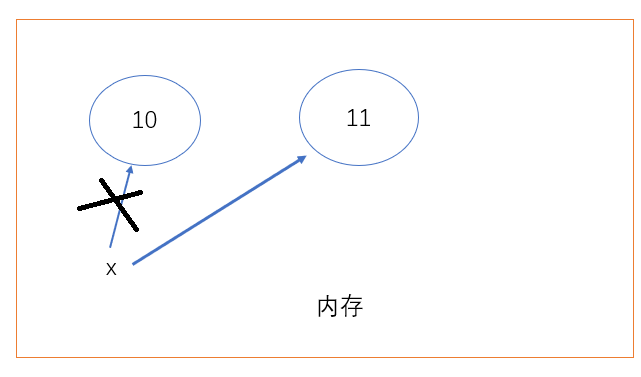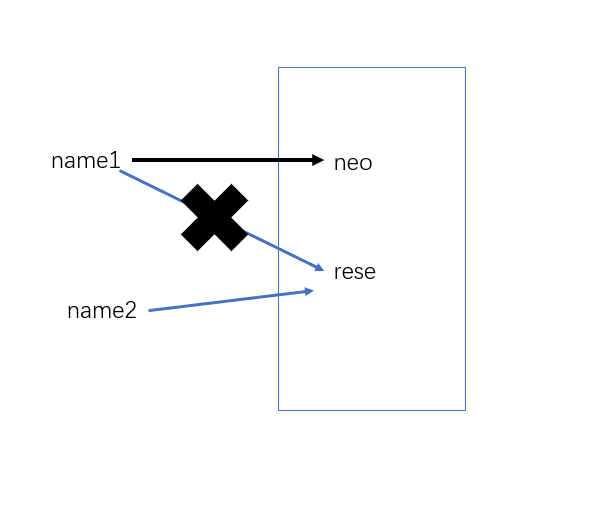变量、数据类型、python内存管理 – Python量化投资

# pycharm快捷键

``````ctrl + c  复制，  默认复制整行
ctrl + v 粘贴
ctrl + x  剪切
ctrl + a   全选
ctrl + z  撤销
ctrl + f  查找
ctrl + shift + z   反撤销
ctrl + d  复制粘贴选中内容，没有选中默认整行
ctrl + y  删除整行
ctrl + backspace   删除一个单词
ctrl + w  选中一个单词
ctrl + shift + r  全局搜索
shift + F10   运行上一个文件
ctrl + shift + F10  运行当前文件
shift + enter  进入下一行
ctrl + /   整体注释
ctrl + alt + l  格式化代码
home  回到行首
end  回到行尾``````

# 变量

## 什么是变量

IPO

I –> input –> 输入（变量）

P –> Process –> 处理

O –> Output –> 输出

## 变量的组成

1. 变量名：具有描述意义； 接受变量值
2. 赋值符号：赋值，把变量值传给变量名
3. 变量值：具体的值

## 变量名的规范

1. 变量名必须要有意义
2. 变量名由数字、字母、下划线组成，不能以数字开头
3. 不能以关键字命名
``['and', 'as', 'assert', 'break', 'class', 'continue', 'def', 'del', 'elif', 'else', 'except', 'exec','finally', 'for', 'from', 'global', 'if', 'import', 'in', 'is', 'lambda', 'not', 'or', 'pass','print', 'raise', 'return', 'try', 'while', 'with', 'yield']``

## 定义变量名的两种方式

``neo_of_name = 'neo'``

``NeoOfName = 'neo'``

# 常量

``````AGE = 19
AGE = AGE + 1   # 这样做就很沙雕了``````

# python内存管理

## python垃圾回收机制

``````x = 10
x = 11````````````name1 = 'rese'
name2 = name1
name1 = 'neo'``````### 引用计数

``````x = 1000   # 1000的引用次数为1
y = 1000    # 1000的引用次数为2
del x   # del删除x，1000的引用次数为1
``````

## 小整数池

``````>>> x = 10
>>> id(10)
140704061711472
>>> y = x
>>> id(y)
140704061711472
>>> z = 10
>>> id(z)
140704061711472   # 内存地址
>>> x = 1000
>>> id(x)
1619602196368  #
>>> x = 1000
>>> id(x)
1619602196496   # 这里内存地址与之前的不同``````

python实现int的时候有个小整数池，这是为了避免因创建相同的值而申请重复的内存空间带来的效率问题。

python解释器会自动定义[-5, 256]之间的 整数池，这是在内存中写死的。这个范围内的整数被全局调用时，永远不会触发垃圾回收机制。

# 变量的三种打印形式

``````age = 20
# 打印值
print(age)
# 打印内存地址
print(id(age))
# 打印数据类型
print(type(age))``````

# 数据类型

## 数字类型

### 整型

``````age = 21
age = int(21)``````

``````x = 2
y = 1
print(x + y)  # 加
print(x - y)  # 减
print(x * y)  # 乘
print(x / y)  # 除
print(x % y)  # 取余
print(x // y) # 取整
print(x ** y)  # 幂运算``````

``````import cmath
print(cmath.sin(10))``````

### 浮点型

``````salary = 3.2
salary = float(3)   # 3.0``````

## 逻辑比较

``````>>> x = 1
>>> y = 2
>>> print(x > y)
False
>>> print(x >= y)
False
>>> print(x < y)
True
>>> print(x <= 1)
True
>>> print(x != y)
True
>>> print(x == y)
False``````

## 字符串

``````name = 'cwz'
name = "cwz's name" ``````

``````str1 = 'neo'
str2 = 'zen'
print(str1 + ' ' + str2)
# 打印结果：neo zen
print(str2 * 10)
# 打印结果：zenzenzenzenzenzenzenzenzenzen``````

# 注释

## 单行注释

``````# 打印12
# print(12)``````
• 解释代码什么意思
• 让后面的代码失效

## 多行注释

``````​'''

​'''``````

https://www.cnblogs.com/setcreed/p/11493835.html

「点点赞赏，手留余香」

还没有人赞赏，快来当第一个赞赏的人吧！
Python
Python
0 条回复 A 作者 M 管理员
所有的伟大，都源于一个勇敢的开始！